What is the number whose is of ?

# What is the number whose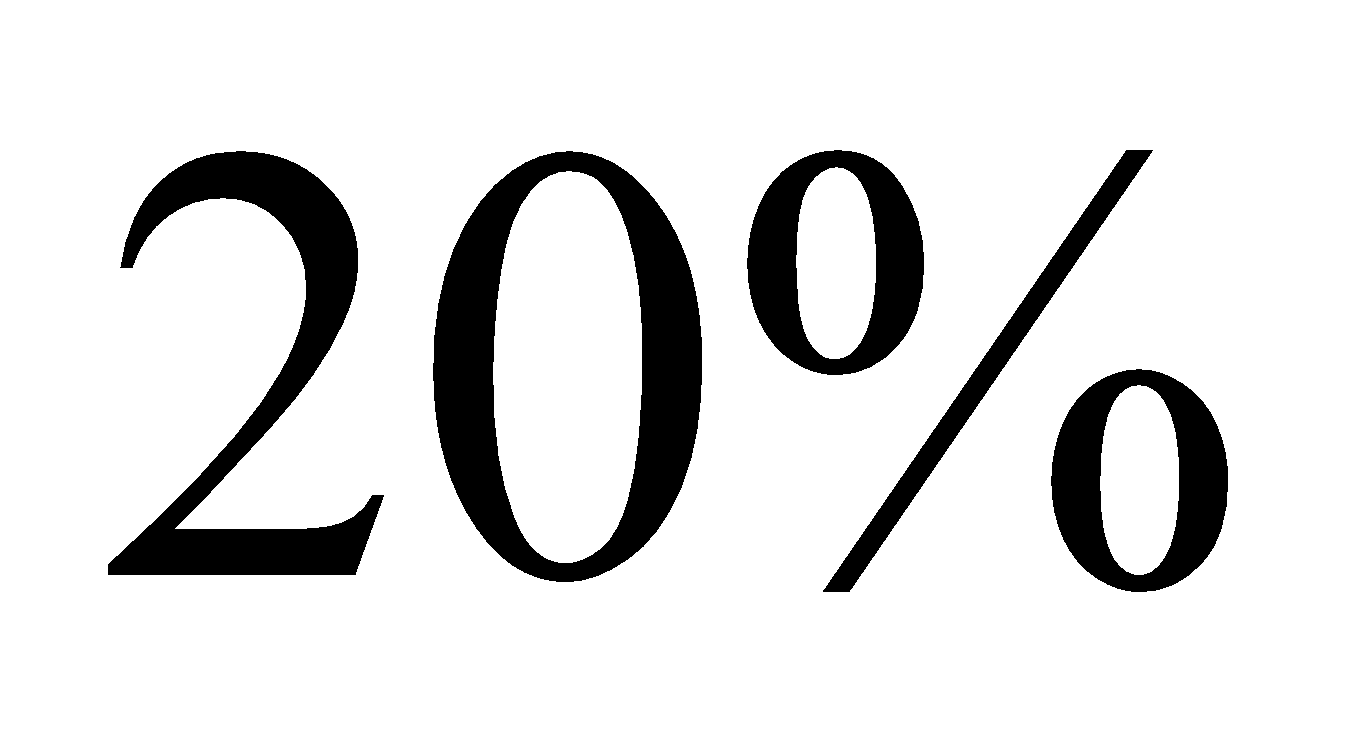is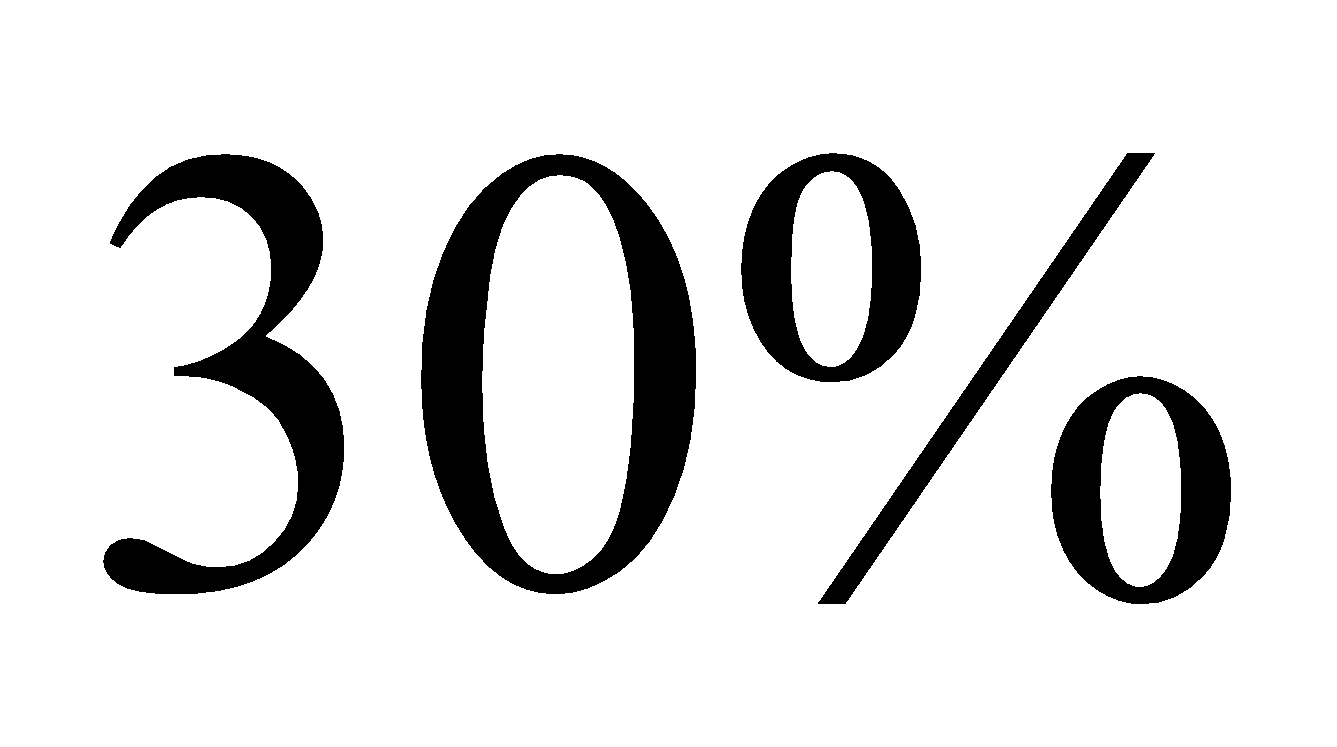of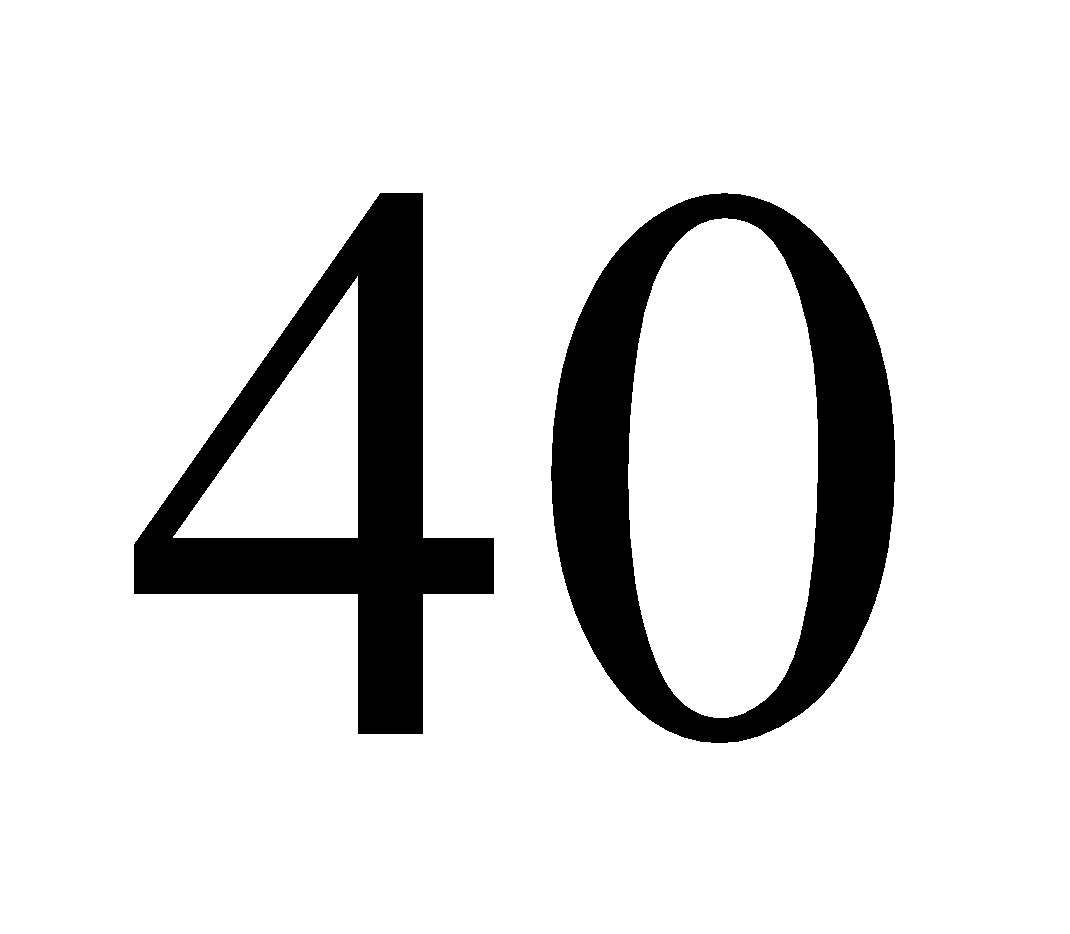?

1. A
90
2. B
80
3. C
60
4. D
50

Fill Out the Form for Expert Academic Guidance!l

+91

Live ClassesBooksTest SeriesSelf Learning

Verify OTP Code (required)

### Solution:

Concept- The most fundamental use of percentages is to contrast one number to another, with the second number rebased to 100.
Consider the number as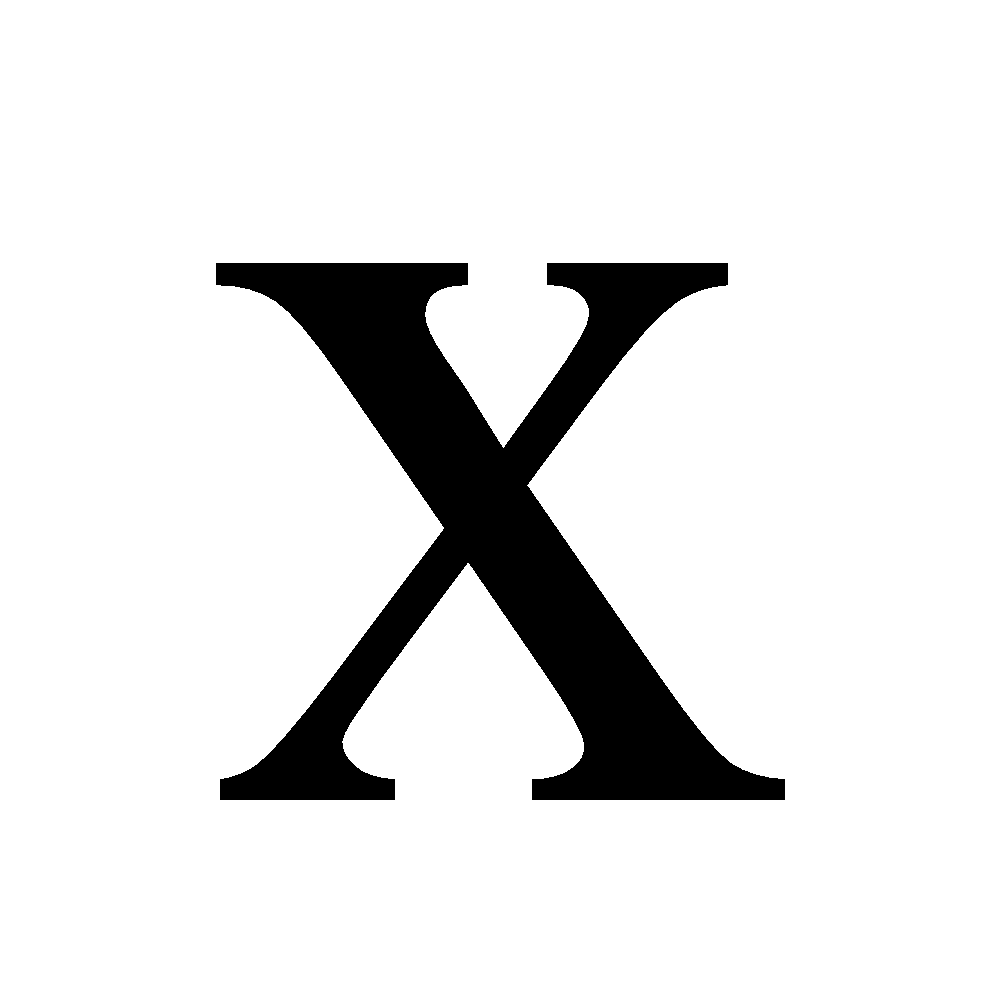.
It is given thatof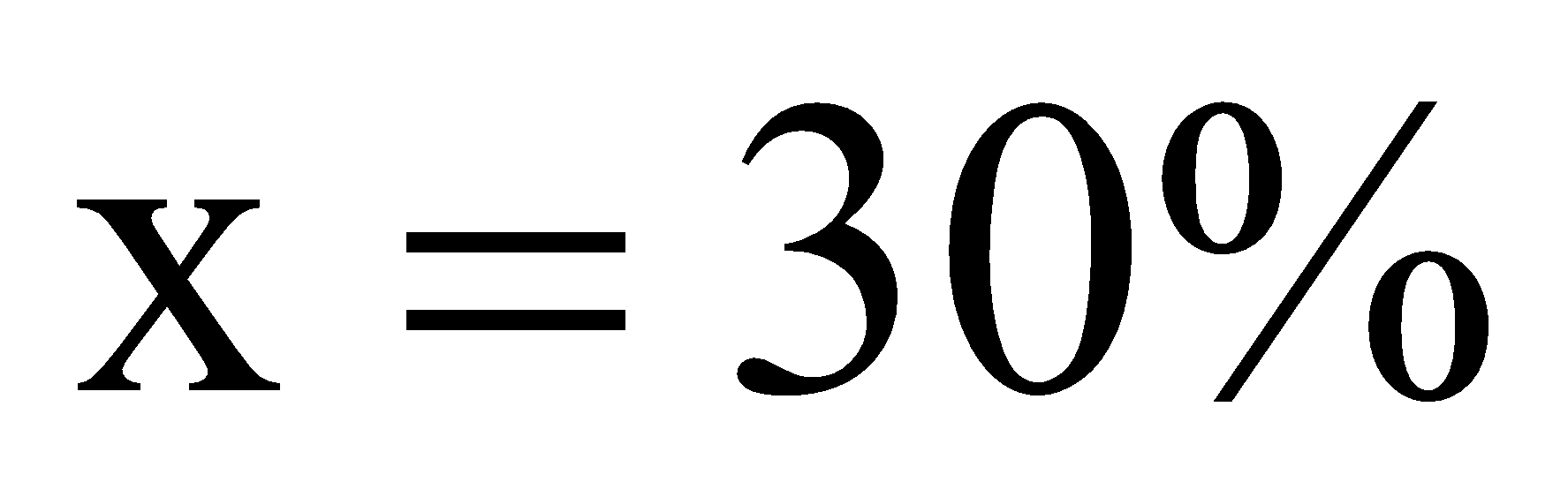of.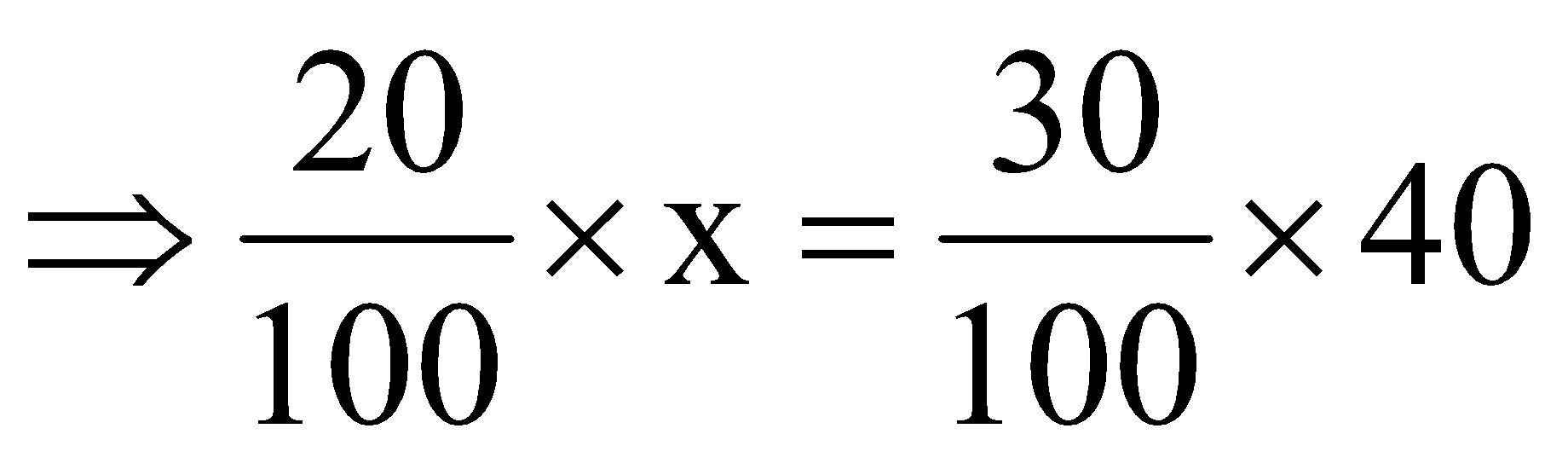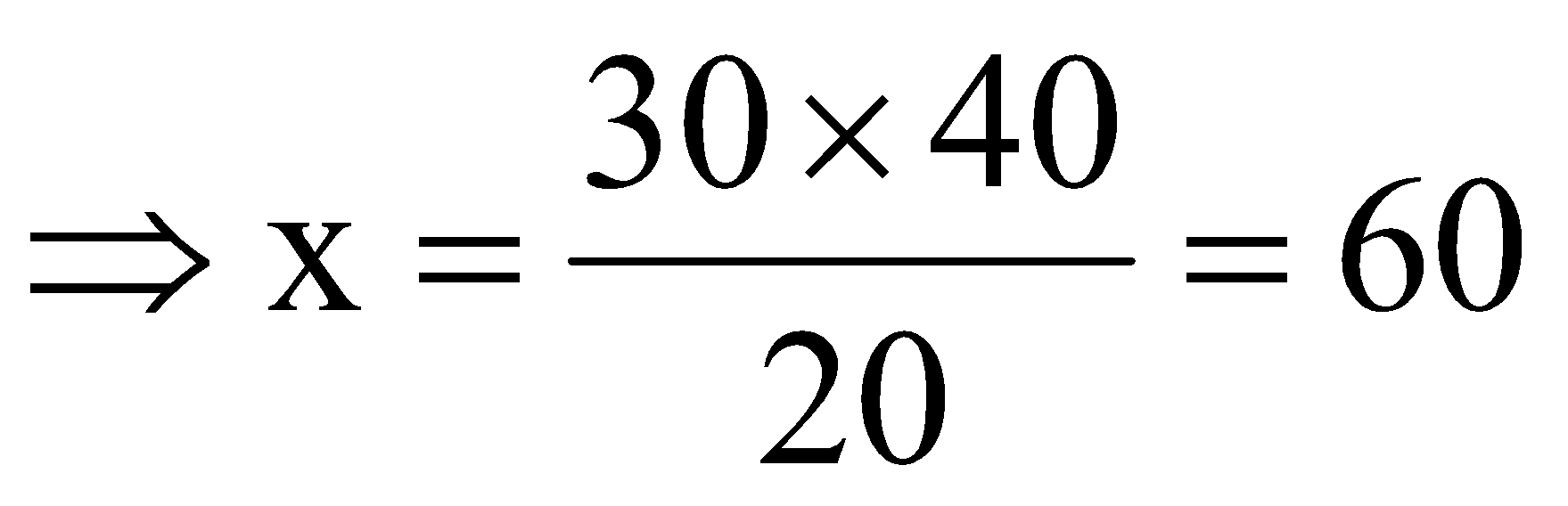Therefore, the number is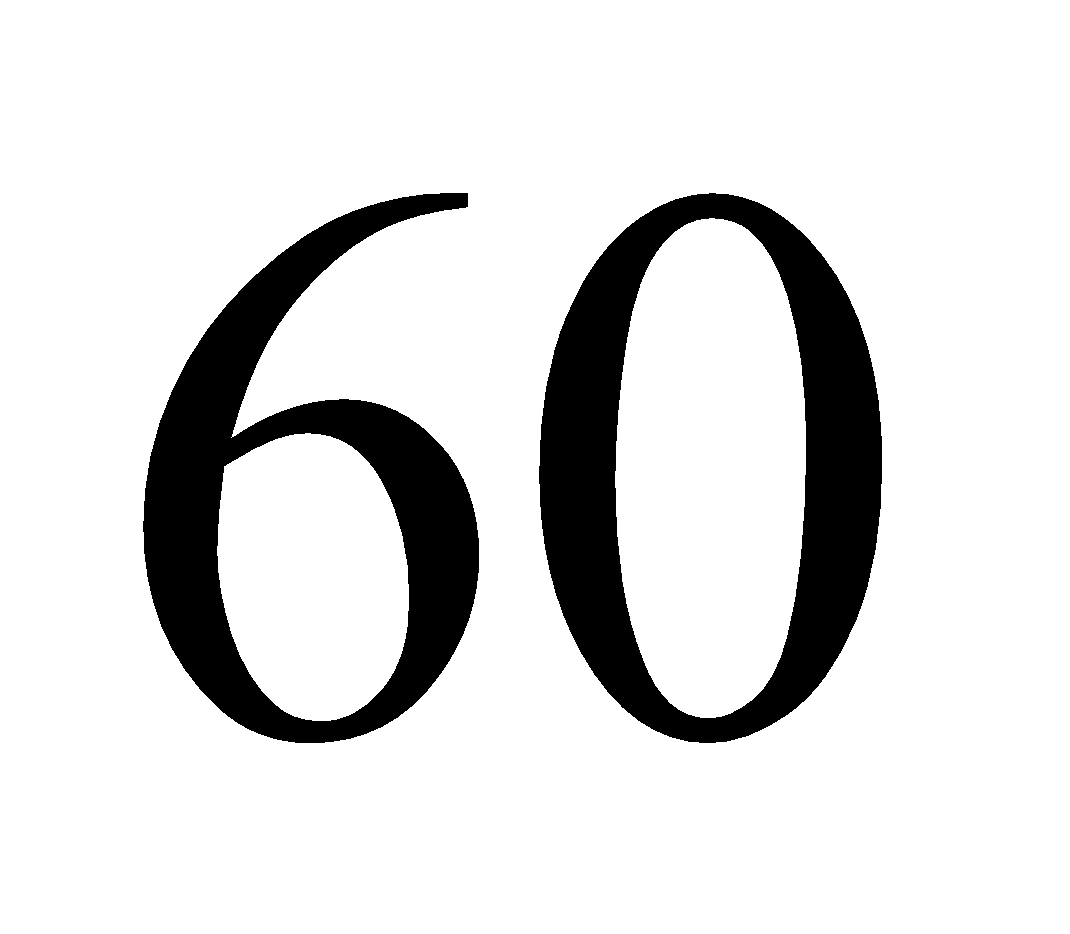.
Hence, the correct answer is option 3) 60.

## Related content

 Area of Square Area of Isosceles Triangle Pythagoras Theorem Triangle Formula Perimeter of Triangle Formula Area Formulae Volume of Cone Formula Matrices and Determinants_mathematics Critical Points Solved Examples Type of relations_mathematics+91

Live ClassesBooksTest SeriesSelf Learning

Verify OTP Code (required)